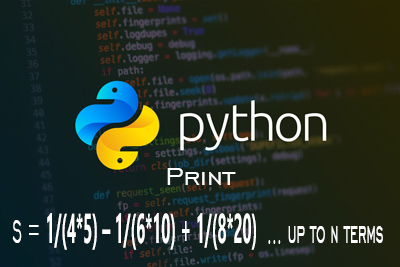Custom Series Print - Python Language
15-10-2020    75 times### Write the Python code to generate the terms of the following series up to n terms (n is user input) using a for loop and find the sum of the terms of the series [you should print the terms of the series exactly as shown below]: S = 1/(4*5) – 1/(6*10) + 1/(8*20) – 1/(10*40) … up to n terms

```N=int(input("Enter Terms : "))
p=S =""
n=4
d=5
for i in range(1,N+1):
if i==1:
p=p + "1/(" + str(n) + "*" + str(d) + ")"
elif i%2==0:
p=p + "-1/(" + str(n) + "*" + str(d) + ")"
else:
p=p + "+1/(" + str(n) + "*" + str(d) + ")"
n=n+2
d=d*2
print(p)

```